# Sample BITSAT Logical Reasoning Test

## 10 Questions MCQ Test BITSAT Mock Tests Series & Past Year Papers | Sample BITSAT Logical Reasoning Test

Description
Attempt Sample BITSAT Logical Reasoning Test | 10 questions in 10 minutes | Mock test for JEE preparation | Free important questions MCQ to study BITSAT Mock Tests Series & Past Year Papers for JEE Exam | Download free PDF with solutions
QUESTION: 1

### Flow : River :: Stagnant : ?

Solution:

As Water of a River flows similarly water of Pool is Stagnant.

QUESTION: 2

### Find the missing character.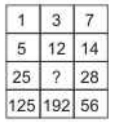Solution:
• The sequence in first column is x 5. Thus, 1 x 5 = 5, 5 x 5 = 25, 25 x 5 = 125.
• The sequence in third column is x 2. Thus, 7 x 2 = 14, 14 x 2 = 28, 28 x 2 = 56.
• The sequence in second column is x 4. Thus, 3 x 4 = 12, 12 x 4 = 48, 48 x 4 = 192.

∴ Missing number = 12 x 4 = 48.

QUESTION: 3

### In following number series, one term is missing find the missing term. 1,5,11,19,29,....,55

Solution:

The correct sequence is + 4, + 6, + 8, + 10, ....
So, next term after 29 = 29 + 12 = 41.
The term after 41 will then be (41 + 14 = 55)
∴ 41 is missing.

QUESTION: 4

Group the following figures into three classes on the basis of identical properties.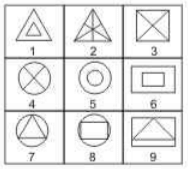Solution:
• 1,5,6 have two similar elements, one inside the other.
• 2,3,4 contain straight lines each dividing the figure into two equal parts.
• 7,8,9 have one element placed inside a different element.
QUESTION: 5

Complete the analogous pair.
Voter : 18 years :: Lok Sabha Member : ?

Solution:

The minimum age required to vote is 18 years similarly for Lok Sabha member it is 25 years.

QUESTION: 6

In this letter series, some of the letters are missing. Choose the correct letter given below -
abca _ bcaab _ ca _ bbc _ a

Solution:

The correct series is abc / aabc / aabbc / aabbcc / a.
∴ Missing letters are abac

QUESTION: 7

Select the correct mirror-image of the Figure (X) from amongst the given alternatives.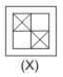Solution:

Because in mirror image right becomes left and left becomes right.

QUESTION: 8

Select the correct mirror-image of the Figure (X) from amongst the given alternatives.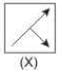Solution:

Because in mirror image right becomes left and left becomes right.

QUESTION: 9

Each of the following questions consists of five Problem figures marked 1,2,3,4 and 5 followed by four Answer figures marked A,B,C and D. Select a figure from the Answer figures which will continue the same series as given in the Problem figures.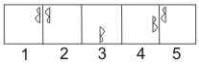Solution:

The symbol gets vertically inverted and laterally inverted alternately. It also moves in anit-clockwise direction through distances equal to two half-sides (of square boundary) and three half-sides alternately.

QUESTION: 10

Each of the following questions consists of five Problem figures marked 1,2,3,4 and 5 followed by four Answer figures marked A,B,C and D. Select a figure from the Answer figures which will continue the same series as given in the Problem figures.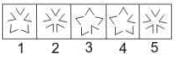Solution:

Two and four 'V' shaped elements get inverted alternately.Use Code STAYHOME200 and get INR 200 additional OFF Use Coupon Code